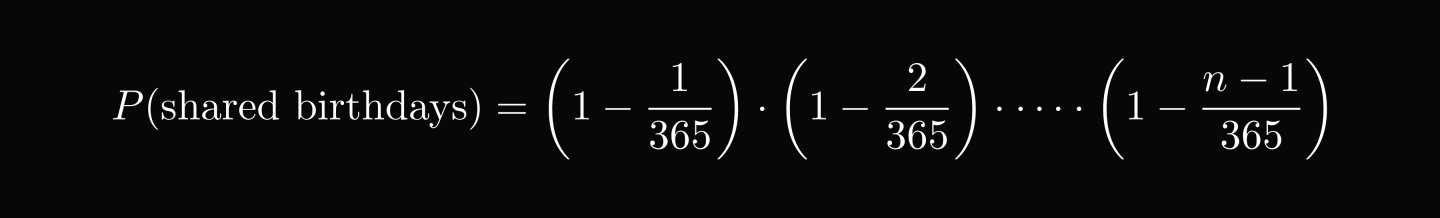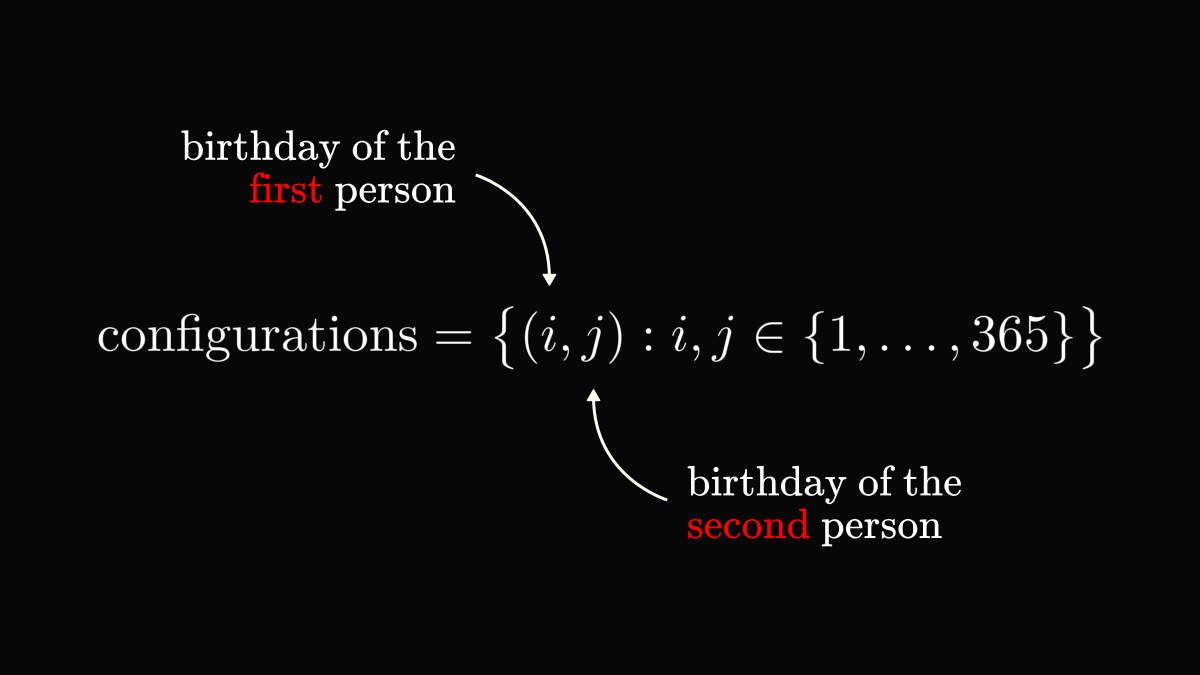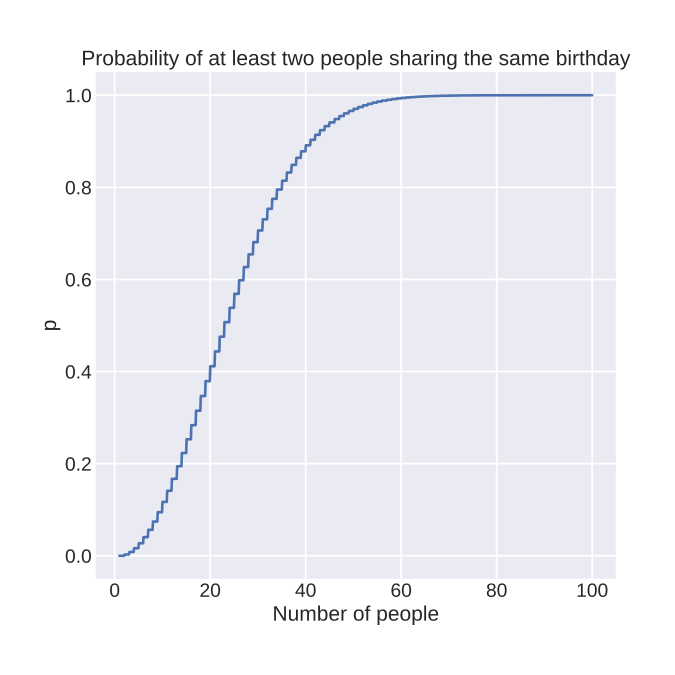There are 25 people in a room. What is the probability that two of them share the same birthday? If you think it is low, you'll be surprised to find out that the actual probability is more than 50%. Here is why!

The usual thinking is if there are 25 people and 365 days in a year, then chances should be roughly 24/365 ≈ 6.5%, and if we have 366 people, then it is guaranteed that two of them share birthdays. However, this is not how probability works.

First, it is much easier to talk about the probability of having no shared birthdays. This is a common trick, often making the calculations much more manageable.

$P(\text{shared birthdays}) = 1 - P(\text{no shared birthdays})$

Let's simplify the problem even more! Given two people, what is the probability of sharing the same birthday? By encoding the birthday with an integer between 1 and 365 and the configurations as tuples, we can easily count their total number. This is how we represent birthdays:

$\text{configurations} = \Big\{(i, j): i, j \in \{1, 2, \dots, 365\}\Big\}.$

How many days can we pick as the 1st element of the tuple? $365$. How many days can we pick as the 2nd element to avoid the birthday collision? $364$.

In total, there are $365 \cdot 364$ ways.To count the number of total configurations, we simply disregard the potential birthday collision. Thus, there are $365 \cdot 365$ possibilities.

Now we can calculate the probability as the ratio of the number of desired outcomes and all possible outcomes:

$\text{probability} = \frac{\# \text{desired outcomes}}{\# \text{all possible outcomes}}.$

In this case, the result is

$P(\text{two people don't share birthdays}) = \frac{365 \cdot 364}{365 \cdot 365}.$

Summing up, the probability of two people sharing the same birthdays is less than one percent.

\begin{align*} P(\text{two people share birthdays}) &= 1 - \frac{365 \cdot 364}{365 \cdot 365} \\ &\approx 0.27 % \end{align*}

What about the general case? Following the same logic, we can solve the general problem, obtaining

$P(\text{there are shared birthdays among } n \text{ people}) = 1 - \frac{365!}{(365 - n)! \cdot 365^n}.$

At $n=23$ people, the probability reaches 50%. At $n=41$, the probability is 90%. Quite surprising, isn't it? If you are curious, this is what the probabilities look like.What can we learn from the birthday problem? That our intuition about probability often fails hard. When asked, most estimate the chances of having a shared birthday among 25 people very low, even though the actual probability is more than 50%.